Complex Calculator Precision 27 1.0.0.9 Complex calculator for scientists, engineers, teachers, and students. Sharewareawkalc 2.2.1 awkalc is a Dashboard widget to evaluate a formula (such as “sin(23)*cos(42)”) using awk. Freeware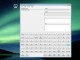Scientific Calculator Decimal 1.0.1.5 Scientific Calculator Decimal for scientists, engineers, teachers, and students. Shareware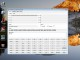Scientific Calculator Precision 54 1.0.1.9 Scientific calculator for scientists, engineers, teachers, and students. Shareware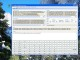Complex Calculator Precision 36 1.0.1.1 Complex number calculator for scientists, engineers, teachers, and students. Shareware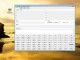Complex Calculator Precision 18 1.0.1.1 Complex number calculator for scientists, engineers, teachers, and students. Shareware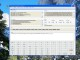Scientific Calculator Precision 63 1.0.1.8 Scientific calculator for scientists, engineers, teachers, and students. Shareware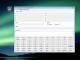College Scientific Calculator 45 1.0.1.8 Scientific calculator for scientists, engineers, teachers, and students. Shareware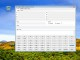College Scientific Calculator 36 1.0.1.8 Scientific calculator for scientists, engineers, teachers, and students. Shareware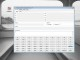College Scientific Calculator 27 1.0.1.8 Scientific calculator for scientists, engineers, teachers, and students. Shareware